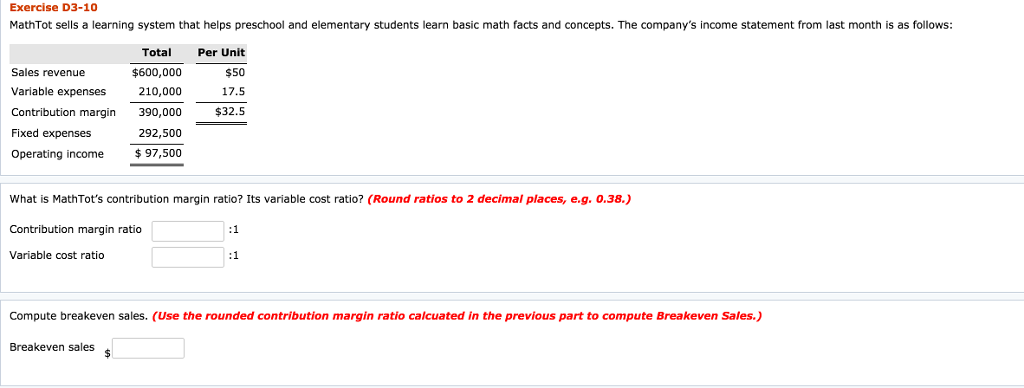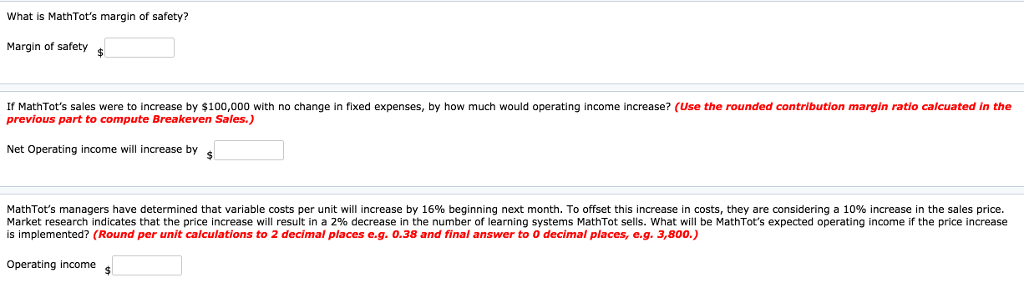# Question & Answer: Exercise D3-10 MathTot sells a learning system that helps preschool and elementary students learn basic math facts and con…..Exercise D3-10 MathTot sells a learning system that helps preschool and elementary students learn basic math facts and concepts. The company’s income statement from last month is as follows Total Per Unit Sales revenue Variable expenses 210,00017.5 Contribution margin 390,000 \$32.5 Fixed expenses Operating income \$97,500 50 \$600,000 292,500 What is MathTot’s contribution margin ratio? Its variable cost ratio? (Round ratios to 2 decimal places, e.g. 0.38.) Contribution margin ratio Variable cost ratio Compute breakeven sales. (Use the rounded contribution margin ratio calcuated in the previous part to compute Breakeven Sales.) Breakeven sales